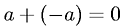Equations > Algebra > Addition/Subtraction > Additive Inverse Property

### Additive Inverse PropertyLatex Code:

MathML Code:

 $a+\left(-a\right)=0$

MathType 5.0: